| Home | TPZero |TPZero User Manual

Tool for Ac=0 Acceptance Sampling PlansRelated Methods Example Input: Consumer's Point: Example Output: Decision Rule: Example Output: Probability Statement of the  Consumer's Point: File Menu Design Menu Help Menu The Right mouse button pop-up menu Entering the Consumer's Point, RQL & Beta Entering the acceptance number for AC>0 Calculated Sample Size, n Points on the OC Curve Producer's Point (AQL,Alpha) Possible Fractions Defective Nomenclature Assumptions and Limitations of TPZero           Range of Input variables           Binomial Distribution Getting Help Buy and Register TPZero How to Contact H & H Servicco Corp. Glossary Credits: TPZero uses the following products:

# What does this tool for Ac = 0 do?

This software tool calculates the sample size for Ac=0 acceptance sampling plans. The user specifies the sampling requirement by entering a Consumer's Point of the oc curve that the sampling plan should have. That is the only input required.

## Related Methods

These zero acceptance number plans are usually called C=0 plans when used for manufacturing and quality control applications, and to "Discovery Sampling" plans when used for auditing financial records.

### Example Input: a Consumer's Point:

RQL = 0.10 fraction defective, Beta = 0.05

n = 28, Ac = 0

### Example Output: of Probability Statement describing the Consumer's Point:

If the true lot fraction defective is 0.10 or more, then the probability that a sample of n =28 will contain 0 defectives is 0.05 or less.

TPZero is a simple tool with only three main menus.

The File menu will print a copy of the report on the default printer, copy it to the Windows clipboard, or exit the program.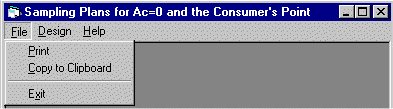The Design menu has only one option -- to design a C=0 sampling plan.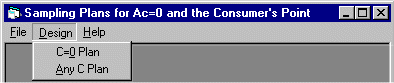The Help menu provides help in using TPZero.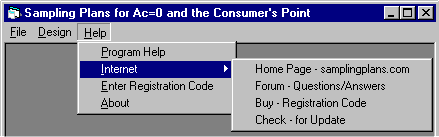## The Right mouse button pop-up menu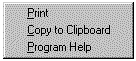# User Interface

## Entering the Consumer's Point, RQL & Beta

You start this form from the Design Menu. [Design][Ac=0].

To calculate the sample size required, you only need to enter two values, RQL and Beta.

To determine a sample size for your application, change the example values of RQL and Beta to your values. Then push the Calculate Button.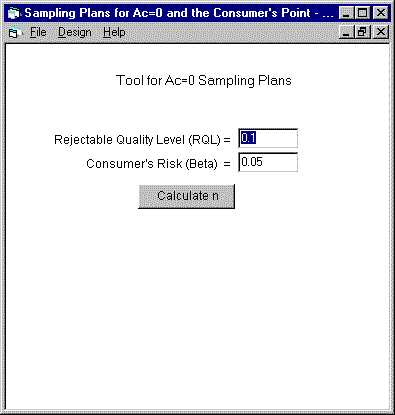## Entering the acceptance number for AC>0

You start this form from the Design Menu. [Design][Ac>0].

As you increase the Acceptance number ( Ac) and do nothing else, the oc curve moves to the right (towards higher fraction defective)

As you move Ac up and RQL down the oc curve becomes steeper, making the plan more discriminating.

## Calculated Sample Size, n

When you push the Calculate Button, TPZero tool calculates the sample size required by the consumer's point ( RQL, Beta).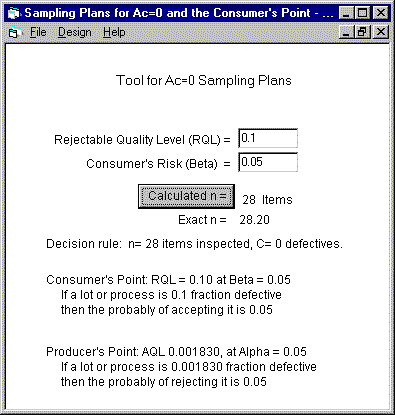### Interpretation of the output:

The Decision Rule· The sample size, n, is rounded to the nearest whole number.· The exact-n displays the value of n that exactly satisfies your consumer's point -- before rounding to the whole number, n. (Shown only for sample size less than 1,000.)· The Ac = 0 decision rule is stated.

### Performance of the Sampling Plan· The exact meaning of your consumer's point is shown as a probability statement.· The producer's point is displayed and interpreted for Alpha = 0.05.

# Using the OC Curve, Plot of Pa versus p'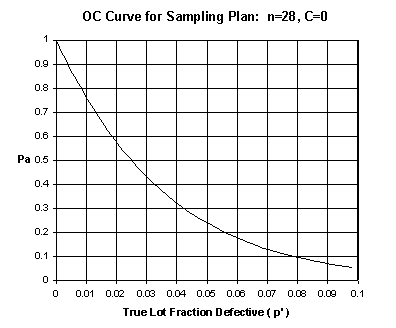This oc curve describes the performance of the decision rule n=28, Ac=0. This plan was designed by specifying the consumer's point: RQL=0.10, Beta=0.05

Every decision rule ( n , Ac ) has an oc curve, which describes the sampling plans' probability of accepting lots having various fractions defective.

The program TPZero displays two points on the oc curve, the Consumer's Point and the Producer's Point.

## Points on the OC Curve

( RQL, Beta) and ( AQL, Alpha) define the consumer's and producer's points on an oc curve. Actually, any point on the oc curve, regardless of what you call it, can be entered for (RQL, Beta). TPZero will calculate the sample size for the one Ac=0 sampling plan that has an oc curve going through that point..

## Producer's Point (AQL,Alpha)

For your information, TPZero shows the producers point, ( AQL and Alpha ) at Alpha = 0.05 for all sampling plans. This second point is not necessary in designing an Ac=0 sampling plan because for a given Ac, you only need to specify one point.

However, knowing the producer's point can be useful if you need to know the lot quality that will have a high probability of acceptance with the plan.

For Alpha = 0.05, Pa = (1-Alpha) = 0.95 (95%)

# Appendix

## Possible Fractions Defective

TPZero does not use lot size, so it can not automatically limit RQL to the possible series of values of lot p'. For any lot containing N items, the possible fractions defective are:

p': 0, 1/N, 2/N, 3/N ....

For example, if N=100, the possible numbers of defective items are:

defectives = 0, 1, 2, 3 ...

So the values of p' are limited to:

p' = 0.00, 0.01, 0.02, 0.03 ...

Consequently, the probability statements will be inexact when RQL is smaller than1/N.

## Nomenclature

Terms and Symbols used by TPZero

 SYMBOL NAME Pa probability of acceptance of a lot. p' fraction defective of a lot. AQL Acceptable Quality Level. RQL Rejectable Quality Level. Alpha risk of rejecting a lot if p'=AQL. Beta risk of accepting a lot if p'=RQL. n Sample Size Exact-n Sample Size before rounding to whole number N Lot Size Ac Acceptance Number, Same as C C Acceptance number, same as Ac X The number of defective items in a sample.

## Assumptions and Limitations of TPZero

### Range of Input variables

RQL: 0.000001 to 0.999999 (decimal fractions)

Beta: 0.000001 to 0.999999 (decimal fractions)

For Ac>0 option. Ac: 0 to 700

### Binomial Distribution

TPZero calculates the sample size using the binomial distribution. The binomial does not use the lot size in calculating n. As such, the binomial is an approximation to the true distribution, the hypergeometric. It is a very accurate approximation and is commonly used when small samples are taken from large lots. As a Rule of Thumb, the binomial distribution is very accurate when N / n =>10. That is, when the lot is 10 times larger than the sample.

## Getting Help

Context sensitive help: help F1

Sampling plan help: www.samplingplans.com

The menu [Help][About] displays the version of TPZero, whether it is registered, and if not, how many days of free usage are left.

## How to Contact H & H Servicco Corp.

 Website: www.samplingplans.com Discussion forum: www.samplingplans.com/forum/forum.htm Email service@samplingplans.com Phone: 651-777-0152 Mail: PO Box 9340 North St Paul, MN 55109-0340 USA

## Glossary

Ac
The Acceptance Number is the maximum allowable number of defective items in the sample and still accept the lot. If the number of defectives in the sample exceeds Ac, then the lot is rejected. The symbols "Ac" and "C" are commonly used to represent the acceptance number.

acceptance number
The acceptance number, symbol=Ac,or C, is the maximum allowable number of defectives in the sample for a lot to be accepted. For the acceptance number of zero, the lot is rejected when a sample contains one or more defective items.

Alpha
The Producer's Risk, Alpha, is the risk of rejecting a good lot, that is, a lot that contains AQL fraction defective.

AQL
The Acceptable Quality Level, AQL, is the fraction defective of a lot that would have a high probability of acceptance. AQL is often associated with 0.95 probability of acceptance ( Pa). AQL and Alpha define the Producer's Point.

Beta
The Consumer's Risk, Beta, is the risk of accepting a rejectable lot, that is, a lot that contains RQL fraction defective. A typical value for the Beta risk is 0.05.

C=0
A C=0 sampling plan is one in which the lot is only accepted if zero defectives occur in the sample. Same as Ac=0.

Consumer's Point
The Consumer's Point is the point on the oc curve defined by RQL on the X-axis and Beta on the Y-axis. Example: beta=0.05 probability of accepting a lot if its fraction defective is RQL=0.10. (10%)

discriminating
The ability of a sampling plan to discriminate (tell the difference) between an AQL lot and RQL lot. A sampling plan can discriminate well when its AQL and RQL are sufficiently close together. If one sampling plan is more discriminating than another, its oc curve is steeper (more vertical).

exact-n
The exact sample size shows the value of n -- before rounding to a whole number -- that exactly satisfies the consumer's point that you entered. The exact n can be useful to know when the sample size is small and the direction of rounding can have economic consequences.

n
n: Lower case n is the symbol for the sample size to be inspected.

N: Upper case N is the symbol for the lot size (Number of items in the lot.)

oc curve
The oc curve (Operating Characteristic Curve) of a sampling plan is a graph of Pa versus p', where Pa = probability of acceptance, p' = true lot fraction defective. The oc curve tells you how the sampling plan will perform in making accept/reject decisions.

p'
p' is the symbol for the fraction of items in a lot that are defective.

Pa
Pa is the probability that a sampling plan will accept a lot. Pa depends on the decision rule (n, C) and the lot's fraction defective.( p)'

probability statement
These two probability statements are produced by TPZero:

The probability of a type II error:

The probability of accepting a lot if it contains RQL fraction defective

The probability of a type I error:
The probability of rejecting a lot if it contains AQL fraction defective.

Producer's Point
The producer's point of the OC Curve is defined by AQL and Alpha.

RQL
The Rejectable Quality Level, RQL, is the fraction defective of a lot that would have a low probability of acceptance. RQL and Beta define the consumer's Point of the oc curve.

Sample Size
The sample size is number of items drawn from the lot for inspection. The symbol for sample size is n, whereas the symbol for the lot size is N.

TPZero
This program is a component of the more comprehensive program "Sampling Plans for Attributes (TP105).

TP105 allows input of BOTH consumer's point and producer's point, or (n, Ac) to calculate the Alpha and Beta risk, or to calculate AQL and RQL.

TP105 also calculates, in addition to the complete oc curve, the AOQ, ARL, ASN, and AFI curves.

TP105 also calculates, for any (n, Ac), the matched sequential sampling plan. Or, from two points on the oc curve, both fixed-n and sequential sampling plans.

## Credits: TPZero uses the following products:

The author of TPZero

Stan Hilliard, H & H Servicco corp.

Visual Basic V6 - Microsoft

To design and compile the program code.

VB Helpwriter

To design the Help system.

FabLock - Teletech Systems

To design the copy protection system

| Home | TPZero |